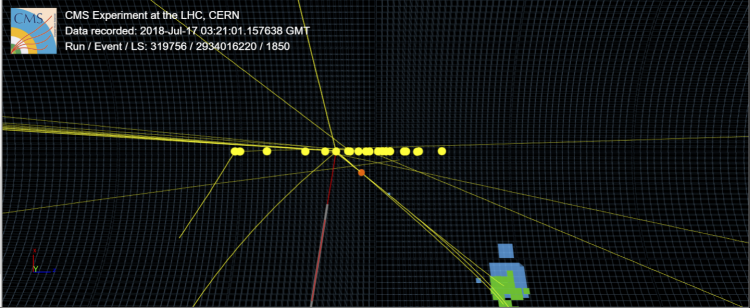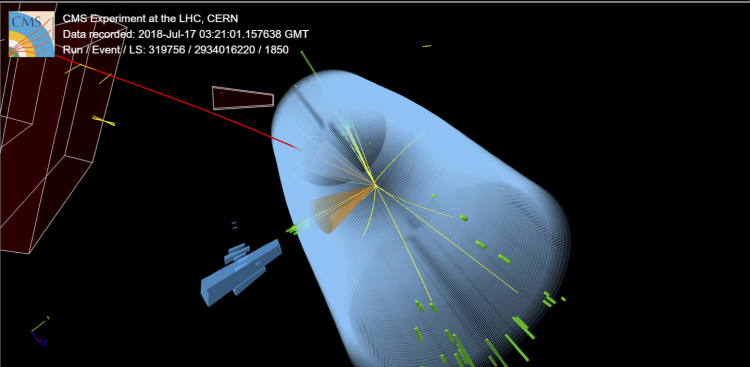•••In 2012 the ATLAS and CMS collaborations discovered a boson with a mass of 125 GeV, i.e. approximately 125 times the mass of the proton, in proton-proton collisions at the CERN LHC. This has marked a milestone in high energy physics, as this particle has all the characteristics of the pivotal Higgs boson predicted more than 50 years earlier. However, many mysteries in the Universe remain unsolved, like the abundant presence of dark matter, the mass of the neutrino, and many others that cannot be explained within the framework of the standard model. These unsolved problems anticipate the existence of other elusive particles.

One possibility to search for hints and traces of new particles is via studies of deviations of the features of the Higgs boson from their expected values. Therefore, since the discovery of this particle, its features have been fiercely reviewed by researchers at ATLAS and CMS. For example, one may wonder how the features of the Higgs boson would look like in a special mirror that would invert left-right, up-down, and charge simultaneously. Such an operation is mathematically referred to as CP conjugation. Any deviation of the Higgs boson image in such a warped mirror would unambiguously signal the presence of physics beyond the standard model.Figure 1: A view of the Higgs boson in a CP mirror (image: DESY/designdoppel)

If the Higgs boson, or more precisely the coupling of the Higgs boson to other particles, does not change in the CP mirror the coupling is said to be CP-even (scalar). If however all coordinates are flipped, just like left and right are flipped in a normal mirror, the coupling is CP-odd (pseudoscalar). The coupling may also be in a mixed state, i.e. containing an even and an odd part and this is quantified mathematically in an angle ϕ. An illustration of the scalar Higgs-boson facing the CP-mirror is depicted in Figure 1.

The CMS Collaboration has made the first measurements of the CP structure of the Higgs coupling to tau leptons, using the data collected in 2016-2018. Once the Higgs boson is produced in the proton collision, it decays in 6% of the cases into a pair of tau leptons that then decay into one or three charged particles and neutrinos. By measuring the angle ϕCP between the planes containing the visible decay objects, CMS could measure for the first time the amount of CP mixture in the Higgs-to-tau coupling.Figure 2: Event display of a proton-proton collision recorded at CMS with a candidate Higgs boson decaying into two tau leptons. The muon track originating from one tau lepton is indicated in red, the three charged hadrons originating from the other decaying tau lepton are indicated with the cone and the blue calorimeter cells.

An example of a selected event with a  candidate Higgs boson decaying to tau leptons is shown in Figure 2, with one of the tau leptons decaying into a muon and two neutrinos, while the other decays into three charged particles and a neutrino. A special property of the tau lepton is that it travels on average 1 mm in the detector before it decays. The zoomed event display depicted in Figure 3 reveals the decay vertex of the candidate tau lepton that decays to three charged particles.Figure 3: Zoomed event display of a proton-proton collision recorded at CMS with a candidate Higgs boson decaying into two tau leptons. The decay location of the tau, which decays into three charged hadrons, is clearly visible and indicated with an orange dot, as well as concurrent proton-proton collisions.

The neutrinos in the tau lepton decays make the measurement very challenging. When the lepton decays to a single charged particle and one or two neutrinos, the reconstruction of the decay plane requires the extrapolation of the charged track back to the proton-proton vertex with a resolution of 20 micrometre; that is approximately half the width of a hair! Higgs bosons are produced very rarely in proton collisions, and then only 6% decay to a tau lepton pair. Many processes that occur much more frequently contribute to a large background and to distinguish the signal events from background processes deep learning algorithms were deployed. To resolve optimally the tiny length scales of the decaying tau leptons and resolve their decay modes special analysis techniques were developed as well.Figure 4: Distribution of the CP angle for the sum of the three most sensitive decay modes. The data are displayed in black, the CP-even prediction is displayed in blue, and the CP-odd hypothesis is in green. The data clearly favour the CP-even hypothesis.

Applying all these techniques to the three years of collision data enables CMS to measure the distribution of the angles between the tau decay planes, which could then be compared to CP-even and CP-odd predictions. These are shown in Figure 4. for the three most sensitive tau decay modes. The data clearly favour the CP-even hypothesis. The CP-odd hypothesis was ruled out with 3.2 standard deviations, or 99.7% probability. It was found that the mixing angle ϕττ is 4±17 degrees, the final result is depicted in Figure 5. The result is consistent with the standard model expectation for a CP-even Higgs boson which is ϕττ = 0. This measurement rules out a part of the phase space of new physics models.Figure 5: A scan of the logarithm of the likelihood of predictions of the mixing angle ϕ ττ.

The uncertainty in this first measurement of the CP structure of the Higgs-tau coupling is largely of statistical nature. This means that with the collection of more collision data during LHC Run 3, as well as during the high-luminosity LHC that will start in 2027, the reflection of the Higgs boson in the CP mirror will emerge with increasing clarity.